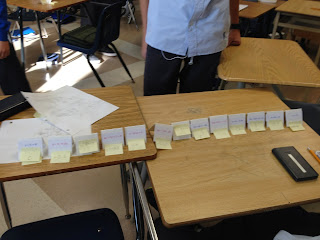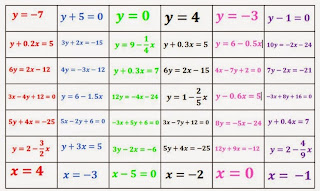## Monday, 25 May 2015

### Increasing Order of SteepnessIn this activity, students will get a set of 7 linear equations and they must start by placing them in increasing order of steepness.  Once their cards are sorted they will be paired with another group with different cards and sort all their cards together as well as answering other questions about their equations.  This is a good activity for rearranging linear equations in the form "y=mx+b" and understanding relative size of slope.

MPM1D
• express the equation of a line in the form y = mx + b, given the form Ax+By+C=0
• identify, through investigation, the equation of a line in any of the forms y=mx+b, Ax + By + C = 0, x = a, y = b
• identify, through investigation with technology, the geometric significance of m and b in the equation y = mx + b
• identify, through investigation, properties of the slopes of lines and line segments
• graph lines by hand, using a variety of techniques
• solve problems requiring the manipulation of expressions arising from applications of percent, ratio, rate, and proportion
MFM2P
• express the equation of a line in the form y = mx + b, given the form Ax+By+C=0.
• identify, through investigation, y = mx + b as a common form for the equation of a straight line, and identify the special cases x = a, y = b
• identify, through investigation with technology, the geometric significance of m and b in the equation y = mx + b
• identify, through investigation, properties of the slopes of lines and line segments
• graph lines by hand, using a variety of techniques• a set of 7 linear equations for each group of 3 - colour copies on cardstock and folded (if you don't have a colour copier, each set of linear equations could be copied on different colour cardstock)
• There are 6 complete sets of cards.  Depending on how big your class is you may need to make 2 complete sets and some groups will have the same set.  We recommend groups no larger than 3.
• 3 blank cards for each set
• a question card for each group
• small stickies for each group (so you can reuse the equation cards - students can write on stickies and attach to the equation card)
• 1 teacher reference sheet - colour copy on cardstock

1. Place students in groups of 3.
2. Give each group a set of linear equation cards, three blank cards, stickies and a question card.
3. Each group starts by putting their set of linear equations in increasing order of steepness (not slope, that will come later).  Tell students that they will be paired up with another group so they may want to include something on their equation card using the stickies.  Use the teacher reference sheet to check the order of steepness quickly.teacher reference sheet
4. When 2 groups are finished pair them up and they must now put all their equations in increasing order of steepness.  Make sure when pairing groups that they have different colour cards. While students are waiting to be paired up with another group they could start on answering the other questions from the question card.
5. The paired groups will then continue answering questions from the question card.
Prompt questions from question card:
1. Arrange the linear equations in increasing order of steepness.
2. Each student in the group must create a new linear equation that can be placed between any two of the original equations.  Place them using the blank cards.
3. Each student in the group chooses one card and creates a line that is parallel and perpendicular to their equation.
4. Each student chooses a different card and creates a line that has the same y-intercept as their equation.
5. Each student chooses a different card and finds the x and y intercepts of that line.
6.  Arrange the linear equations in increasing order of slope.  Would the line up look the same?  Discuss with your group.
Note:  In some cases, some groups did not pass putting the equations in increasing order of steepness so they were never paired up with another group.  Not all groups finished all the prompting questions. As an extension, you may consider having all the groups put their equations on a single continuum.

The video, below, is only visible in the WECDSB domain. That is, only teachers in our school board can see the video if they are logged into their MyTools2Go accounts.

Increasing Order of Steepness complete (pdf) (doc)

Did you use this activity? Do you have a way to make it better? If so tell us in the comment section. Thanks